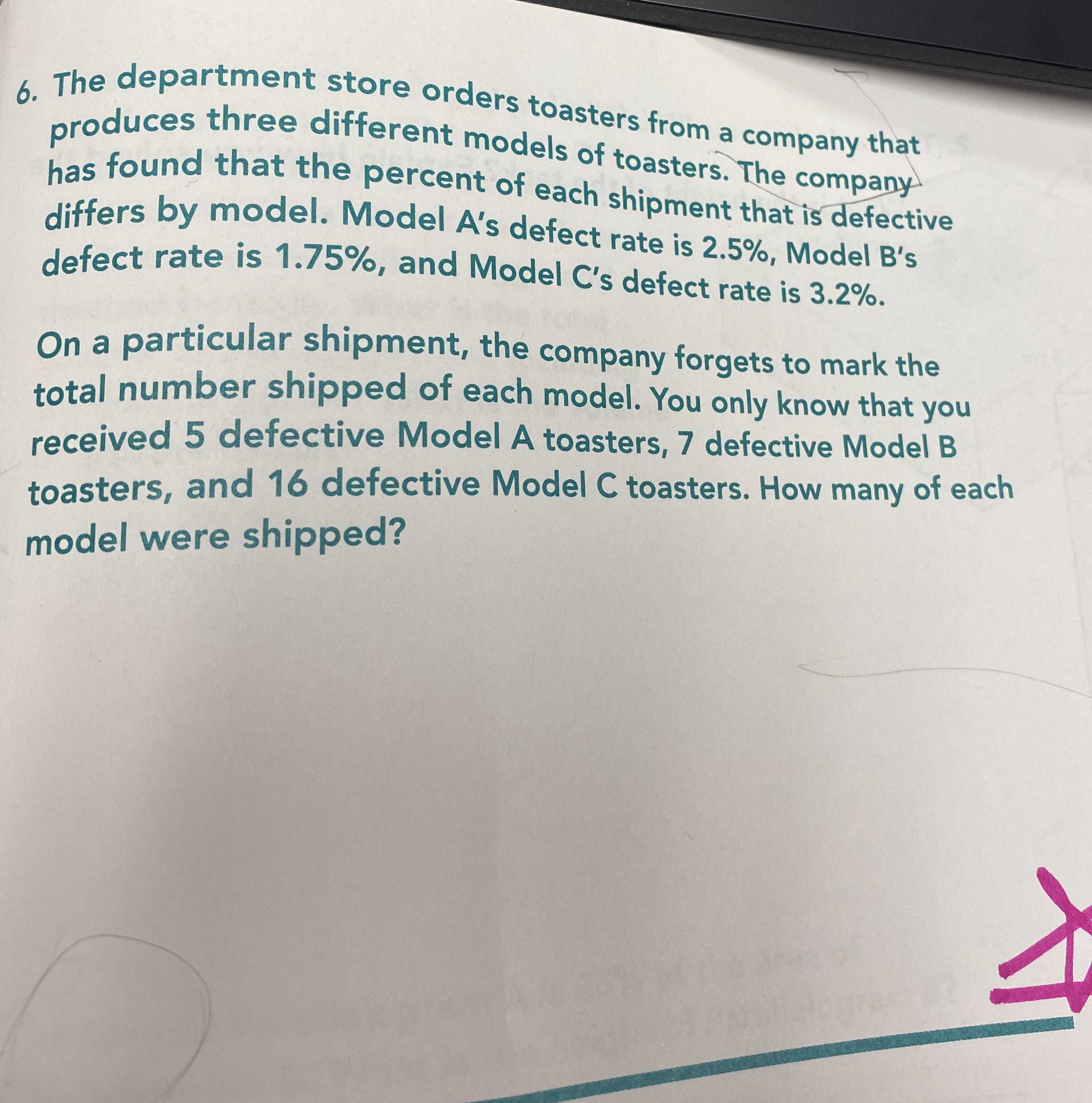### ¿Todavía tienes preguntas de matemáticas?

Pregunte a nuestros tutores expertos
Algebra
Pregunta6. The department store orders toasters from a company that produces three different models of toasters. The company has found that the percent of each shipment that is defective differs by model. Model A's defect rate is $$2.5 \% ,$$ Model B's defect rate is $$1.75 \% ,$$ and Model C's defect rate is $$3.2 \% .$$ On a particular shipment, the company forgets to mark the total number shipped of each model. You only know that you received $$5$$ defective Model A toasters, $$7$$ defective Model B toasters, and $$16$$ defective Model C toasters. How many of each model were shipped?